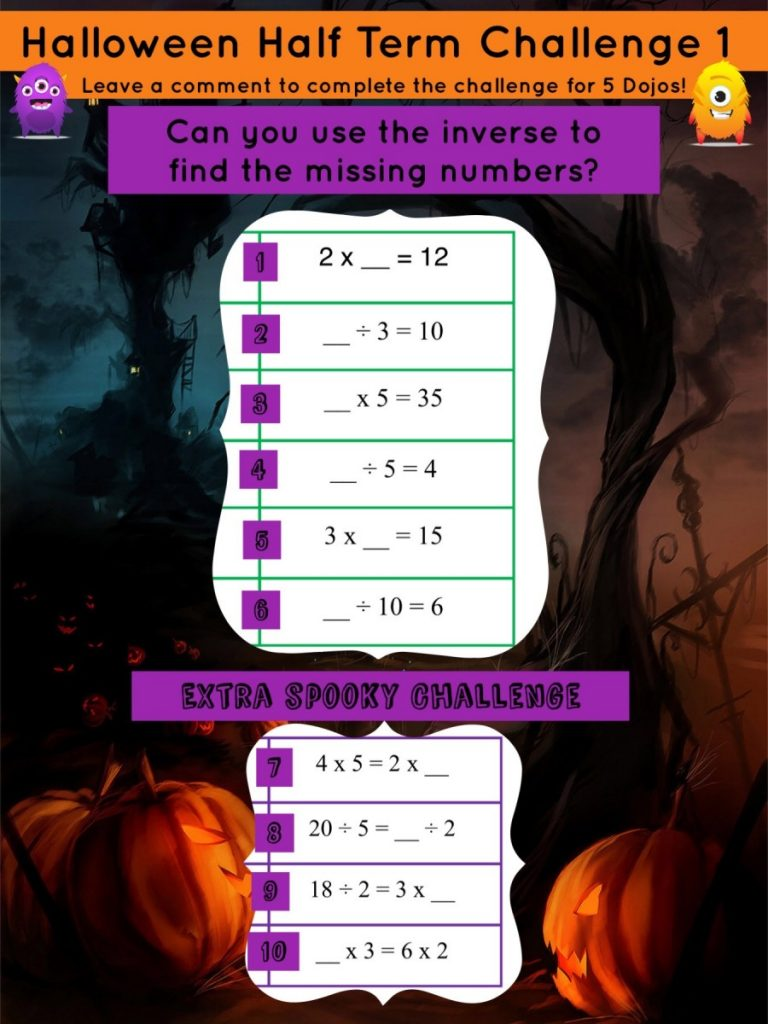### Halloween Half Term Challenge 1Zara

2×6=12,
30÷3=10,
7×5=35,
20÷5=4,
3×5=15,
60÷10=6,
4×5=2×10,
20÷5=8÷2
18÷2=3×3,
4×3=2×6.

Eben

1 = 6 6 = 60
2 = 30 7 = 10
3 = 7 8 = 8
4 = 20 9 = 3
5 = 5 10 = 4

Amilia

1. 2×6=12
2.30 divided by 3 equals
3×7=35
4. 20 divided by 5 equals 4
5.3×5=15
6.60 divided by 10 = 6
7.4×5=20 2×10=20
8.20 divided by 5=4 8 divided by 2=4
9.18 divided by 2=9 3×3=9
10.3×4=12 6×2=12

1) 6
2)30
3)7
4)20
5)5
6)60
7)10
8)8
9)3
10) 4
Homework sheet in maddison book bag.

Selma

2×6=12
30÷3=10
7×5=35
20÷5=4
3×5=15
60÷10=6
4×5=2×10
20÷5=8÷2
18÷2=3×3
4×3=6×2Mrs Lowrie

Year 2 Teacher

I’m Mrs Lowrie! I have worked at WCPS for over 10 years and it is one of my favourite places to be. I love teaching the amazing learners of Worsbrough Common Primary School but I also love to learn new things myself - just like you! At home, I have a lovely little boy and I enjoy spending time with my family and friends and going for long walks.